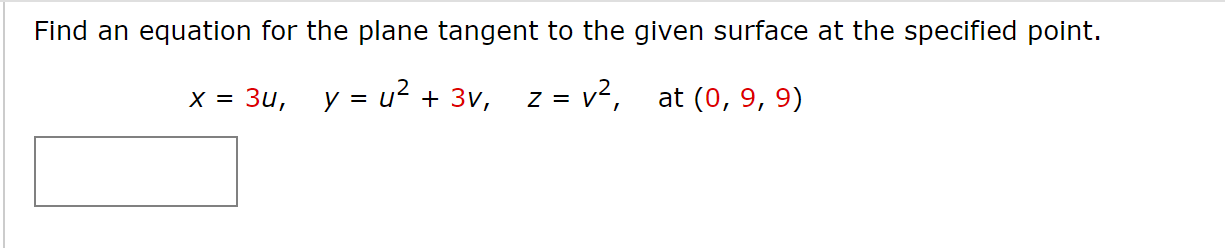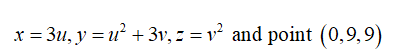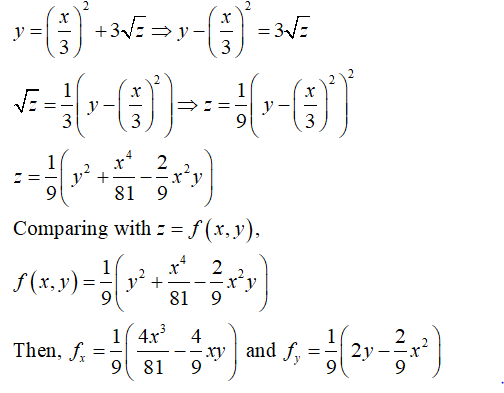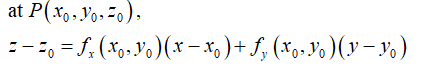# Find an equation for the plane tangent to the given surface at the specified point.z = v²,y = u? + 3v,at (0, 9, 9)Зи,

Question
1 viewshelp_outlineImage TranscriptioncloseFind an equation for the plane tangent to the given surface at the specified point. z = v², y = u? + 3v, at (0, 9, 9) Зи, fullscreen
check_circle

Step 1

Given thatStep 2

Equation of surface can be written as,Step 3

Equation of the tangent plane to the surface z = f (x,y):...

### Want to see the full answer?

See Solution

#### Want to see this answer and more?

Solutions are written by subject experts who are available 24/7. Questions are typically answered within 1 hour.*

See Solution
*Response times may vary by subject and question.
Tagged in

### Math